## 4.2 GSM half rate speech decoder

06.203GPPHalf rate speech transcodingTS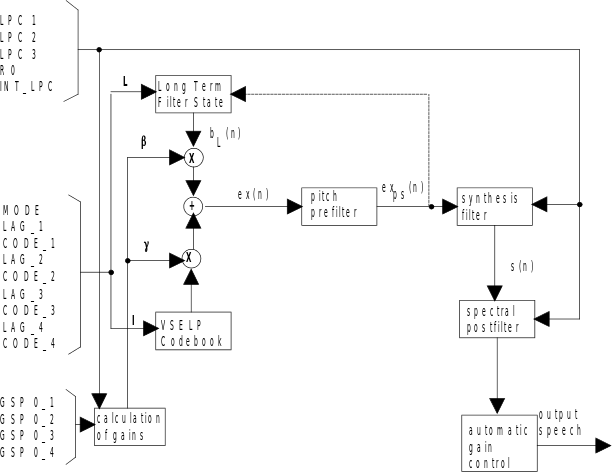Figure 5: The GSM half rate speech decoder for MODE = 1, 2 or 3

A block diagram of the GSM half rate speech decoder for MODE=1, 2 or 3 is given in figure 5. The speech decoder creates the combined excitation signal, ex(n), from the long term filter state and the VSELP codevector. For MODE=0, the long term filter state is replaced by another VSELP codebook and the pitch prefilter is not used. The combined excitation is then processed by an adaptive pitch prefilter and gain. The prefiltered excitation is applied to the LPC synthesis filter. After reconstructing the speech signal with the synthesis filter, an adaptive spectral postfilter is applied followed by an automatic gain control which is the final processing step in the speech decoder.

### 4.2.1 Excitation generation

The combined excitation, ex(n), shall be computed as shown in equation (127).

The combined excitation, ex(n), is filtered by the synthesis filter to generate the speech signal. The synthesis filter is a tenth order all pole filter. The filter coefficients for the subframe are the i’s defined in subclause 4.1.6. The filter coefficients will change from subframe to subframe. The filter state shall be preserved from subframe to subframe. A direct form filter shall be used for the synthesis filter.

Given ex(n) as the input, exp(n), the pitch prefiltered output, is defined by:

; for 0 £ n £ Ns‑1 (150)

where: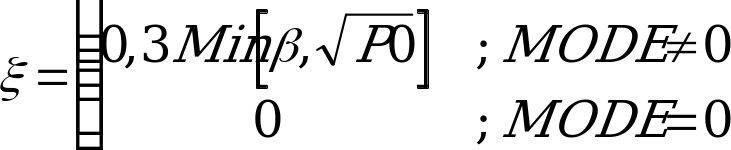(151)

Since L can be fractional in value, an interpolating filter is used. This is the same interpolating filter which is used for the open loop lag search. A gain scale factor is computed and is used to scale the pitch prefiltered excitation, prior to applying it to the LPC synthesis filter. Pscale, the gain scale factor, is: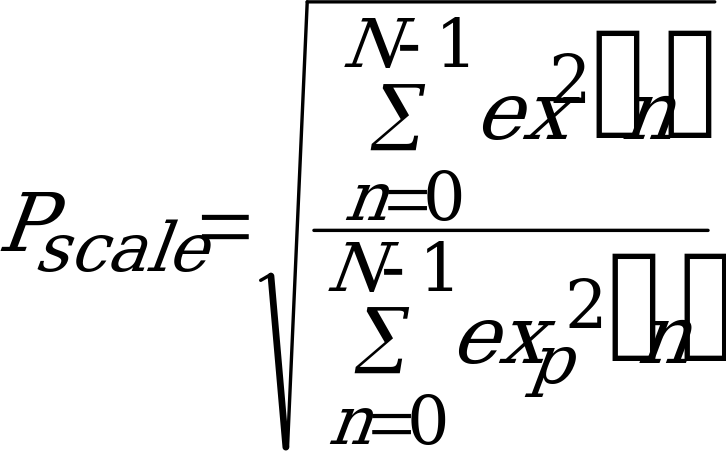(152)

Thus exps(n), the gain corrected pitch prefiltered excitation which drives the LPC synthesis filter, is given by:;for 0 £ n £ Ns‑1 (153)

### 4.2.3 Synthesis Filter

A direct form synthesis filter is used: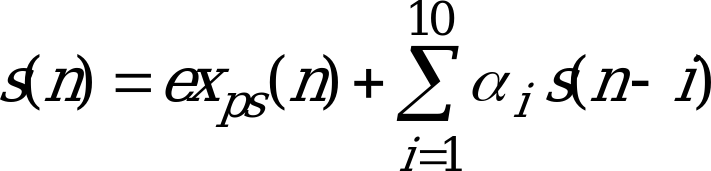, 0 £ n £ Ns‑1 (154)

The perceptual quality of the synthetic speech is enhanced by using an adaptive postfilter as the final processing step. The general form of the postfilter is given by: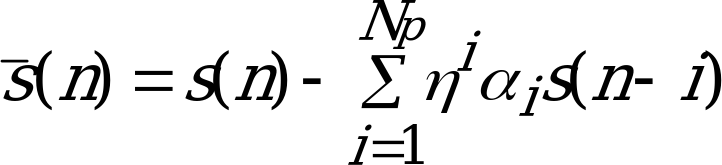,0 £ n £ Ns‑1 (155)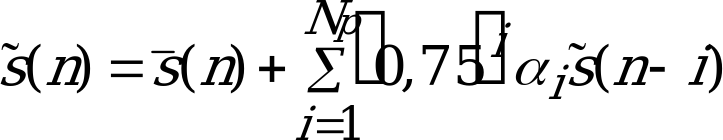,0 £ n £ Ns‑1 (156)0 £ n £ Ns‑1 (157)

The adaptive spectral postfilter numerator polynomial equation (155) is replaced by a spectrally smoothed version of the adaptive spectral postfilter denominator polynomial equation (156). To derive the coefficients of the numerator polynomial, the denominator polynomial coefficients are converted to the autocorrelation coefficients R(i). The SST bandwidth expansion function is then applied to the autocorrelation sequence,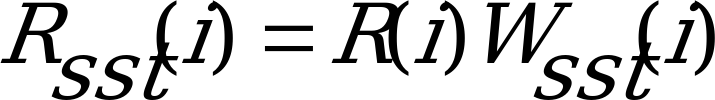, 0 £ i £ Np (158)

and the numerator polynomial coefficients are calculated from the modified autocorrelation sequence via the AFLAT recursion.

From Rsst(i) the reflection coefficients which define the combined spectrally noise weighted synthesis filter are computed using the AFLAT recursion once per frame.

 STEP 1 Define the initial conditions for the AFLAT recursion: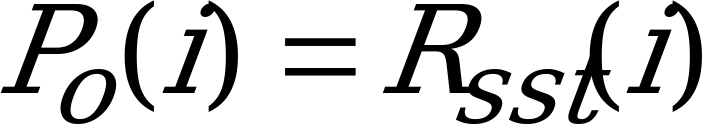,0 £ i £ Np (159)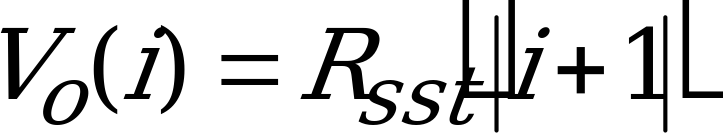,1-Np £ i £ Np‑1 (160) STEP 2 Initialize j, the index of the lattice stage, to point to the first lattice stage:j=1 (161) STEP 3 Compute rj, the j-th reflection coefficient, using: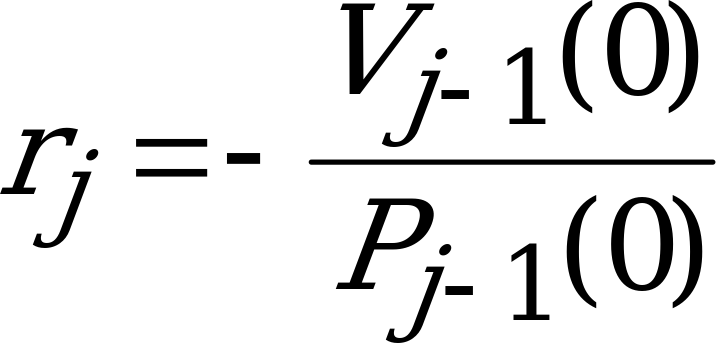(162) STEP 4 Given rj, update the values of Vj and Pj arrays using: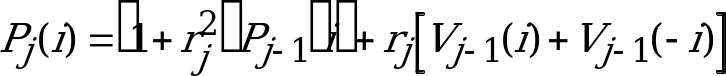.0 £ i £ Np – j – 1 (163)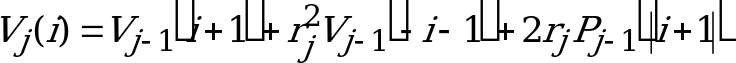,1+j-Np£i£Np-j‑1 (164) STEP 5 Increment j:j = j +1 STEP 6 If j £ Np go to step 3, otherwise all Np reflection coefficients have been obtained. STEP 7 The reflection coefficients, rj, are then converted toi, the direct-form LP filter coefficients for use in the adaptive spectral postfilter numerator polynomial.

The resultant adaptive spectral postfilter is derived from equations 155, 156 and 157: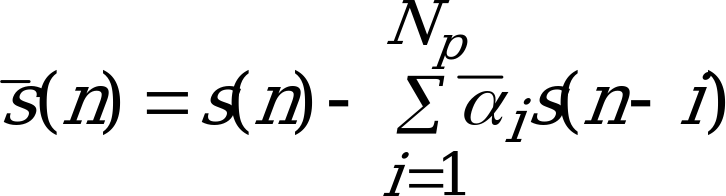,0 £ n £ Ns‑1 (165)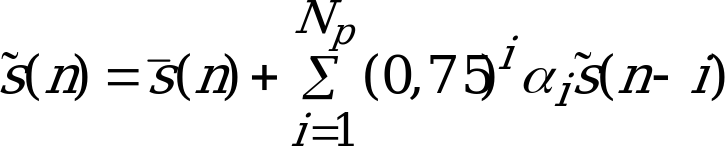,0 £ n £ Ns‑1 (166),0 £ n £ Ns‑1 (167)

In order to reduce the computations needed to compute the spectrally smoothed numerator coefficients, the spectral smoothing operation is performed once per frame on the denominator coefficients corresponding to the uninterpolated coefficients. This will yield the coefficients for the numerator of the spectral postfilter for subframe four. The numerator coefficients for subframes one, two, and three are interpolated using the same interpolation scheme that is used for the LPC synthesis coefficients (see subclause 4.1.6).

As in the case of the pitch prefilter, a means of automatic gain control is needed to ensure unity gain through the spectral postfilter. A scale factor, Sscale, is given by:

S’scale(n) = (0,9875 S’scale(n‑1) ) + (0,0125 Sscale ) (168)

Scale factor, Sscale, is the square root of the ratio of the input signal energy to the output signal energy over the subframe.

The output of the spectral postfilter is then multiplied by S’scale as the last step in reconstructing the speech signal in the speech decoder.

### 4.2.5 Updating decoder states

The long term predictor state, r(n), is updated by:

r(n) = r(n+40) for ‑146 £ n £‑41 (169)

r(n) = ex(n+40) for ‑40 £ n £ ‑1 (170)